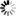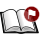Normal view

# The effect of the partial redistribution of spectral line formation K. E. Rangarajan [Ph.D Thesis]

Material type:TextPublication details: Bangalore Indian Institute of Astrophysics 1987Description: 114pOnline resources: Dissertation note: Doctor of Philosophy Indian Institute of Astrophysics, Bangalore 1987 Summary: The main effort of this study is concentrated on ascertaining the role of partial redistribution(PRD> functions in the process of spectral line formation. The effects of angle averaged R I' R II' RIIIa nd R V redistribution with isotropic phase function are studied. We have compared these results with those obtained using complete redistribution (CRD) . Transfer equation with plane parallel geometry is solved using the Discrete space theory technique of Grant and Hunt (1969) . Various types of boundary conditions considered. The following results are the new and important conclusions of this study. In this study, we find that for a purely scattering optically thick medium, RI function produces deeper absorption profile compared to other functions. The redistribution function R II is more coherent than Rv and R v is more coherent than R: u:x in the wings. The more non-coherent the redistribution function is, the higher would be the emergent intensity in the Doppler core. If thermal sources are present in the medium and if there is incident radiation on the lower boundary, all the redistribution functions give the same intensity, in the core. But in the wings, the more non-coherent the redistribution is, the higher would be the intensity. The presence of continuous opacity makes the spectral lines appear weak. Their effects are more pronounced compared to that of the thermal sources in the medium and are present for any type of redistribution mechanism. In high optical depth situations, the R type of redistribution allows the photons to diffuse to the line centre and increase the intensity there. In the third chapter, coherent and non-coherent electron scattering combined with complete and partial redistribution by atoms are studied for some parameterized models. Since this problem is characterized by two frequency scales, one for the atoms and the other for the electrons, two types of frequency quadrature are required to cover the effect of both the processes. Though the basic equations are solved within the framework of Discrete space theory, the frequency quadrature points, normalization, segmenting the problem into core and wing regions and the iteration procedure all follow that of Auer and Mihalas (1968). We obtain the following new result: If the coherent electron scattering is the only continuous opacity source, we find that the more non-coherent the redistribution by atoms is, the higher the value of the mean intensity in the wings for optically thick media. The non-coherent electron scattering combined with PRD fills up the core and hence one gets higher fluxes in the core compared to coherent electron scattering_ We investigate the deviation of absorption and emission profiles from each other for a two level atom with angle avera9~d redistribution functions. The corr~ct expression for the source function derived by Baschek,Mihalas and Oxenius (1981) is used to solve consistently for the emission profile and the radiation field. From this study, we get the following new and important results: The absorption and emission profiles do not deviate from each other in the Doppler core for any redistribution function even if the stimulated emission term is important. The devition of absorption and emission profiles in the wing is more for coherent type of redistribution function. R xxx redistribution gives identical absorption and emission profiles and so one can approximate RIII by CRD for all practical purposes. The effects of small macroscopic velocity fields on Ca II Hand K lines are given in chapter 5. The atomic model chosen contains the lower most five levels and the continuum. radiative transfer equation and statistical equilibrium equations are solved simultaneously using equivalent two level atom method. A schematic chromospheric type of atmosphere is considered. The emergent profiles are calculated for the systematic expanding velocities 0.0, 0.5 and 1.0 (velocities at the outer boundaries expressed in mean thermal units). We obtain the following significant results: Even though the velocities are small, they seem to affect the shapes of the H and K profiles considerably. A single peak emission instead of a double• peaked emission is obtained for the K line with v ~ 1 and p = 0.79. ( ~ = cos8 , 8 is the angle of the ray to the normal at the surface.). The small velocities do not affect the infrared triplet lines significantly.
Tags from this library: No tags from this library for this title.
Star ratingsAverage rating: 0.0 (0 votes)
Holdings
Item type Current library Shelving location Call number Status Date due BarcodeThesis & Dissertations IIA Library-Bangalore General Stacks 043:52/RAN (Browse shelf(Opens below)) Available 10991

Doctor of Philosophy Indian Institute of Astrophysics, Bangalore 1987

The main effort of this study is concentrated on
ascertaining the role of partial redistribution(PRD> functions
in the process of spectral line formation. The effects of
angle averaged R I' R II' RIIIa nd R V
redistribution with
isotropic phase function are studied. We have compared these
results with those obtained using complete redistribution
(CRD) . Transfer equation with plane parallel geometry is
solved using the Discrete space theory technique of Grant and
Hunt (1969) . Various types of boundary conditions
considered. The following results are the new and important
conclusions of this study.
In this study, we find that for a purely scattering
optically thick medium, RI function produces deeper absorption
profile compared to other functions. The redistribution
function R II is more coherent than Rv and R v is more coherent
than R: u:x in the wings. The more non-coherent the
redistribution function is, the higher would be the emergent
intensity in the Doppler core. If thermal sources are present
in the medium and if there is incident radiation on the lower
boundary, all the redistribution functions give the same
intensity, in the core. But in the wings, the more non-coherent
the redistribution is, the higher would be the intensity. The
presence of continuous opacity makes the spectral lines appear
weak. Their effects are more pronounced compared to that of the
thermal sources in the medium and are present for any type of
redistribution mechanism. In high optical depth situations,
the R type of redistribution allows the photons to diffuse
to the line centre and increase the intensity there.
In the third chapter, coherent and non-coherent
electron scattering combined with complete and partial
redistribution by atoms are studied for some parameterized
models. Since this problem is characterized by two frequency
scales, one for the atoms and the other for the electrons, two
types of frequency quadrature are required to cover the effect
of both the processes. Though the basic equations are solved
within the framework of Discrete space theory, the frequency
quadrature points, normalization, segmenting the problem into
core and wing regions and the iteration procedure all follow
that of Auer and Mihalas (1968). We obtain the following new
result: If the coherent electron scattering is the only
continuous opacity source, we find that the more non-coherent
the redistribution by atoms is, the higher the value of the
mean intensity in the wings for optically thick media. The
non-coherent electron scattering combined with PRD fills up
the core and hence one gets higher fluxes in the core compared
to coherent electron scattering_
We investigate the deviation of absorption and
emission profiles from each other for a two level atom with
angle avera9~d redistribution functions. The corr~ct
expression for the source function derived by Baschek,Mihalas
and Oxenius (1981) is used to solve consistently for the
emission profile and the radiation field. From this study, we
get the following new and important results: The absorption
and emission profiles do not deviate from each other in the
Doppler core for any redistribution function even if the
stimulated emission term is important. The devition of
absorption and emission profiles in the wing is more for
coherent type of redistribution function. R xxx redistribution
gives identical absorption and emission profiles and so one
can approximate RIII by CRD for all practical purposes.
The effects of small macroscopic velocity fields on
Ca II Hand K lines are given in chapter 5. The atomic model
chosen contains the lower most five levels and the continuum.
radiative transfer equation and statistical equilibrium
equations are solved simultaneously using equivalent two level
atom method. A schematic chromospheric type of atmosphere is
considered. The emergent profiles are calculated for the
systematic expanding velocities 0.0, 0.5 and 1.0 (velocities
at the outer boundaries expressed in mean thermal units). We
obtain the following significant results: Even though the
velocities are small, they seem to affect the shapes of the H
and K profiles considerably. A single peak emission instead of
a double• peaked emission is obtained for the K line with v
~ 1 and p = 0.79. ( ~ = cos8 , 8 is the angle of the ray to
the normal at the surface.). The small velocities do not
affect the infrared triplet lines significantly.

There are no comments on this title.